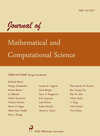Journal of mathematical and computational science (JMCS) is a peer-reviewed open access international journal, which is aimed to provide a publication forum for important research in different areas covering all aspects of mathematical and computational science, such as numerical analysis, optimization, linear and nonlinear programming, theory of computation, control theory, theory of algorithms, computational logic, applied combinatorics, coding theory, cryptograhics, fuzzy theory, differential equations, algebra and number theory. This journal will accept high quality articles containing original research results and survey articles of exceptional merit.### Vol 10, No 2 (2020)

This issue is currently in progress but contains articles that are final and fully citable.

 R. Divya, M. Jayalakshmi Neighborhood pseudo chromatic polynomial of a path J. Math. Comput. Sci., 10 (2020), 219-235 PDF

 Rupesh T. More, Shridhar C. Patekar, Ashish P. Nawghare Study of Ulam Hyers stability of integrodifferential equations with nonlocal condition in Banach spaces J. Math. Comput. Sci., 10 (2020), 236-247 PDF

 Sudev Naduvath, Johan Kok On certain topological indices of signed graphs J. Math. Comput. Sci., 10 (2020), 248-261 PDF

 D. D. Pawar, W. D. Patil, D. K. Raut Numerical solution of fractional order mathematical model of drug resistant tuberculosis with two line treatment J. Math. Comput. Sci., 10 (2020), 262-276 PDF

 B. Reddappa, A. Parandhama, S. Sreenadh Peristaltic transport of conducting Williamson fluid in a porous channel J. Math. Comput. Sci., 10 (2020), 277-288 PDF

 K. Shakila, R. Selvi A note on fuzzy soft paraopen sets and maps in fuzzy soft topological spaces J. Math. Comput. Sci., 10 (2020), 289-308 PDF

 S. Jenifer Wency, A. Lourdusamy Several result on SD-prime cordial graphs J. Math. Comput. Sci., 10 (2020), 309-315 PDF

 S.C. Li, M. Zhou, P.S. Zheng, X.X. Dong, Q.M. Gui Analysis of seepage pressure in dual-porosity reservoir under elastic boundary J. Math. Comput. Sci., 10 (2020), 316-338 PDF

 D. Susha, Manju John Soft valuation on a generalized soft lattice J. Math. Comput. Sci., 10 (2020), 339-358 PDF

 Vijay Vir Singh, Praveen Kumar Poonia, Ameer Hassan Adbullahi Performance analysis of a complex repairable system with two subsystems in series configuration with an imperfect switch J. Math. Comput. Sci., 10 (2020), 359-383 PDF

 Shalini Tomar, Nawneet Hooda Quadratic (s1,s2)-functional inequality in fuzzy normed space J. Math. Comput. Sci., 10 (2020), 384-393 PDF

 Johan Kok, Sudev Naduvath On the rainbow neighbourhood number of set-graphs J. Math. Comput. Sci., 10 (2020), 394-402 PDF

J. Math. Comput. Sci.

ISSN: 1927-5307

Editorial Office: jmcs@scik.org